#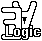## Overview:

 Logic is the basis of rationality and a foundation for mathematics, science and technology. Particularly for information technologies.
 WHAT IS LOGIC? Answered from two different perspective (and more to come). for techies an introduction to symbolic logic, the science of deduction for thinkers a philosophical introduction to logic applications An attempt to separate out the use of logic from study of or research in logic.
 first-order logic First-order logic, comprising the propositional and predicate calculi, is the most widely studied and used. combinators,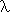-calculi and type systems Three interrelated topics at the heart of logic and computer science. mathematical logic Mathematical Logic (as an academic subject) is the branch of mathematics which is concerned with logic. It has four traditional branches.
 set theory and other abstract ontologies history pieces of the past logic & computing logic & maths logic & philosophy perspectives on the scope of logic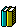## What is Logic?

### (for techies)

 A formal language, given a precisely defined syntax and semantics, becomes a logic when rules for correct reasoning in the language are formally described.
 Introduction Logic is for constructing proofs, which give us reliable confirmation of the truth of the proven proposition. Formal Languages A language is formal if the syntax of the language is defined with sufficient precision that a computer could be programmed to check whether any particular sentence belongs to the language.
 Formal Logics An inference rule is a method of deriving conclusions from premises. When inference rules for a formal language are codified it becomes a formal logic. Varieties of Logic There are many different kinds of logical system within which proofs can be conducted.## What is Logic?

### (for thinkers)

 We present the view that logic is the study of necessary truths and of formal systems for deriving them, explore what this means for philosophy, and note some of the alternative viewpoints.
 Necessity and Contingency To understand logic it is first necessary to grasp the important distinction between contingent propositions, which might or might not be true, and necessary propositions, which could not be false. support for the distinction against the distinction Logic is the study of necessary truths and of systematic methods for clearly expressing and rigorously demonstrating such truths. why this definition? other definitions Logic and Analytic Philosophy Because the methods of analytic philosophy are mainly a priori philosophy is concerned with logic not only as a subject matter but as an important contribution to the methods of philosophy. Analytic Philosophy IS Logic Analytic Philosophy IS NOT Logic

## ApplicationsofAn attempt to separate out the use of logic from study of or research in logic.
 The logic we are considering here is just formal, symbolic or mathematical logic, and my purpose is to separate out the use of logic from study of or research in logic (though research into something other than logic would count).
 A majority of people who take an interest in logic prefer to study logic, to advocate the use of logic, or even to build tools to support the use of logic, rather than to actually use logic. It is also not uncommon to find great aspirations, but little substance, even among great philosophers such as Descartes, Leibniz and Hobbes.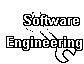Many different aspects of software engineering, from database management, through programming language design to artificial intelligence have benefitted from the discoveries of modern logic.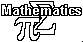Mathematics, always a deductive science, was the target application for the modern revolution in logic. Its has been transformed by modern logic, and can expect more revolution to come.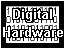Digital hardware is called "logic", its built up from electronic devices selected to behave like boolean operations, and it is the most pervasive and simple application of logic.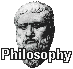Many philosophers have sought to emulate the deductive rigour of mathematics, but none have succeeded. Though Russell and the logical positivists hoped modern logic would make this possible, success remains illusory.## First-Order Logic

 First-order logic, comprising the propositional and predicate calculi, is the most widely studied and used.
 Propositional Logic Propositional logic is concerned with the logic of truth functional sentential or propositional operators such as and, or and not. We provide three accounts with increasing precision and rigour, the informal, semi-formal, and formal descriptions.
 Predicate Logic Extends propositional logic with a treatment of the "quantifiers" all and some. Our informal, semi-formal, and formal descriptions of first-order prdicate logic provide increasing degrees of precision and rigour.

## Mathematical Logic

 Mathematical Logic (as an academic subject) is the branch of mathematics which is concerned with logic. It has four traditional branches.
 proof theory Proof theory has its roots in the beginnings of mathematical logic, in David Hilbert's great programme of metamathematics. It is concerned with the theory of logical systems as syntactic objects. It is also the home of Gödel's famous incompleteness theorems which showed the impossibility of Hilbert's central objective, and also of formal systems for constructive mathematics, an approach radically opposed to Hilbert's philosophy. model theory Model theory begins with the semantics of first order logic, in which we learn what first-order logic is about, viz: models. Model theory concerns the kinds of thing which can be expressed in first-order and other logics, understood through their impact on the models of the theories which can be expressed in these logics.
 set theory Mathematics provides tools to science for building mathematical models of the world around us. Set theory provides the building blocks from which the models are made. Research in set theory is based on first order axiomatisations of the theory, such as ZF. computability theory Originally called "recursion theory" after Kleene's recursive functions, this branch of mathematical logic began with the work of Alonzo Church and Alan Turing who first demonstrated that there are unsolvable problems. This discovery lead to the development of a substantial body of theory about degrees of recursive unsolvability in which unsolvable problems are arranged into a heirarchy. The emergence of the digital computer also gave motivation to the study of computational complexity, which concerns how quickly the answers to various solvable problems can be computed.
some more recent aspects of mathematical logic
 categorical logic Since the initial development of set theory advances have been made in the treatment of abstract and fundamental notions in mathematics resulting first in category theory, and then in the category theoretic approaches to set theory in the form of topos theory. This has lead to a new perspective on logic and the foundations of mathematics in which logic is studied through abstract mathematics, very remote from its origins in syntactic systems. domain theory Domain theory is a topic in theoretical computer science concerned with the semantics of programming languages. Because of its heavy use of abstract mathematics (e.g. category theory) and its focus on the semantic aspects of formal languages this field lies with categorical logic in an important area of interaction between mathematical logic and theoretical computing.
 computational logic Computational logic lies on the boundary between mathematical logic and that part of computer science concerned with the implementation of software concerned with machine assisted reasoning. foundations of mathematics Though foundational motivations played a prime role in the inception of mathematical logic, it has only recently been promoted as a distinct field. Prominent in recent academic research on the foundations of mathematics has been reverse mathematics, which seems concerned to classify mathematical results (particularly in combinatorial mathematics) according to the strength of the set theoretic axioms required to prove them.

## Combinators,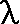-calculi and Type SystemsThree interrelated topics at the heart of logic and computer science.
 The-Calculus A pure calculus of functional abstraction and function application, with applications throughout logic and computer science. Types The-calculus is good tool for exploring type systems, invaluable both in the foundations of mathematics and for practical programming languages. Pure Type Systems A further generalisation and systematic presentation of the class of type systems found in the-cube.
 Combinators Combinatory logic shows that bound variables can be eliminated without loss of expressiveness. It has applications both in the foundations of mathematics and in the implementation of functional programming languages. Programming Languages The connections between the lambda-calculus and programming languages are diverse and pervasive. Type systems are an important aspect of programming language design.
 The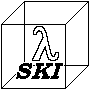-cube A graphical presentation of the relationship between combinatory logic, lambda calculi and related logical systems. The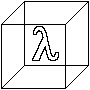-cube A graphical presentation of the relationship between various typed-calculi, illuminating the structure of Coquand's Calculus of Constructions.

## Set Theory and Other Ontologies#### The Iterative Conception of Set

My attempt to explain the fundamental ontological intuition on which modern mathematics is founded.

#### Higher Order Set Theory

Doing set theory in higher order logic is cool (and convenient). HOST is my presently preferred pragmatic set theoretic foundation system. See also ZFC in HOL (an earlier very similar story).

#### Alternative Abstract Ontologies

I have some notes on new classical logical foundation systems, including Pure Functions. Also some ancient writings with ontological discussions.

## Some Historical Bits

#### Logical Revolutionspast, present and future

A story about some sea changes in which our view of logic has played a role.

#### The History of Formal Logicby John Harrison

A section from his paper on Formalised Mathematics.

## Logic and Computing#### Proof and Computation

We mention some of the variety of connections between proof and computation.

#### Automation of Reasoning

Getting computers to find the proofs for us, and using logic for the Mechanisation of Mathematics.

#### Effective Procedures

a definition of this concept fundamental both to logic and computing.

A way of implementing proof checkers/generators for logical systems, also useful for precise specification of logics.
Example LCF style logic specifications: propositional logic, predicate logic.

## Logic and MathematicsThe Formalisation of Mathematics A sketch of the period from about 1821 to 1908, which begins with the publication of Cauchy's Cours d'analyse algebraic and concludes with the publication in 1908 of Russell's Theory of Types and Zermelo's axiomatisation of set theory. Formality and Rigour in 20th Century Mathematics A few words about two issues which have remained outstanding since the formalisation of mathematics, both of which relate in different ways to the complexity of the resulting systems. Formalised Mathematics: Advocacy of the conduct of mathematics in a fully formalised way using computerised tools, including The QED Manifesto and John Harrison's paper on Formalised Mathematics.
 The Foundations of Mathematics - What is a foundation? What alternatives are there? Logicism - the thesis that mathematics is logic. The Logical Foundations of Mathematics Thumbnail sketch of the history of logical foundations for mathematics. Formal Foundation Systems for Mathematics A taxonomy of formal systems distinguishing logics from other formal systems and foundation systems from other logics.

## Logic and Philosophy:

 A philosophical approach to the understanding of logic, its significance and its applications.
 What is Logic? (philosophical) Logic is the study of necessary truths and of systematic methods for clearly expressing and rigorously demonstrating such truths. What is Logic? (symbolic) An introduction to symbolic logic. Definitions of Logic Some ways of defining "Logic" are introduced and their merits discussed. Formal Analyticity An attempt to sharpen up the identification of logical necessity and analyticity.
 Necessity and Contingency We consider the notions of necessary and contingent partly through consideration of possible worlds, in order to explicate logical truth. Logicism (mathematical) Logicism is a philosophical theory about the status of mathematical truths, to wit, that they are logically necessary or analytic. Logicism (philosophical) Philosophical Logicism revisits Bertrand Russell's dream of a philosophy made logically rigorous, taking advantage of a century of development in logic, mathematics and computing.

## Perspectives

#### Perspectives on The Scope of Logic

Logic as technology, logic for Artificial Intelligence, general philosophical and specifically epistemological viewpoints.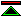©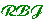created 1994/09/22 modified 2009/03/31